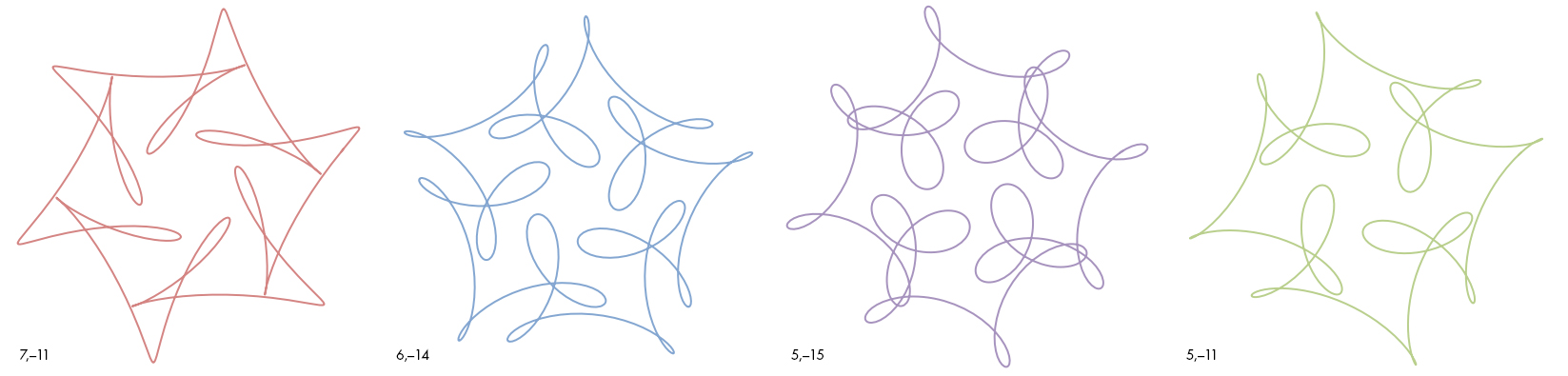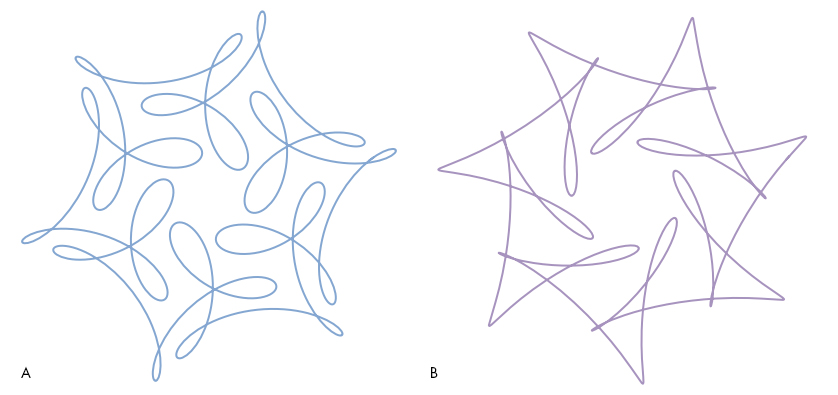# ‘Creating Art With Mathematics’

Our Insights puzzle this month challenged Quanta readers to create pleasing symmetrical curves using mathematical expressions after solving a couple of problems, which were intended to help them divine the principles behind examples given by Frank Farris in his book Creating Symmetry: The Artful Mathematics of Wallpaper Patterns (Princeton University Press, 2015). As usual, our readers rose to the occasion, clearly explaining how the mathematics works to generate the symmetries, and then producing some breathtaking curves of their own.

Here are the questions and their solutions:

Question 1:

Look at the family of symmetrical curves below, one of which is featured in Farris’ book as a “mystery curve”:Each of these curves has an ordered pair of numbers associated with it, shown at the lower left of the curve. These two numbers determine the type of symmetry and complexity exhibited by the curve, and a simple mathematical relationship between the two numbers is required to generate the curve’s pleasant symmetry. In the second question below, we’ll give you the precise way these numbers are used to generate their curve. Without looking ahead, ponder these numbers and try to get an intuitive sense of how the curves change as the numbers do. Then look at curves A and B below and see if you can match them to the correct pairs of numbers, from the 10 choices given. Give your reasons for choosing your particular pairs and describe what you think the relationship is between the two numbers.Choices:

1. (5, –19) 2. (6, –19) 3. (7, –17) 4. (7, –12) 5. (7, –19)
6. (8, –20) 7. (8, –12) 8. (8, –13) 9. (8, –19) 10. (6, –24)

With careful observation it is not hard to establish a simple intuitive correlation between each curve and its pair of numbers, without any reference to the actual equations that generated them. From left to right, our example curves have sixfold, fivefold, fourfold and fourfold symmetry, while the first numbers associated with them are 7, 6, 5 and 5, respectively.

More here.